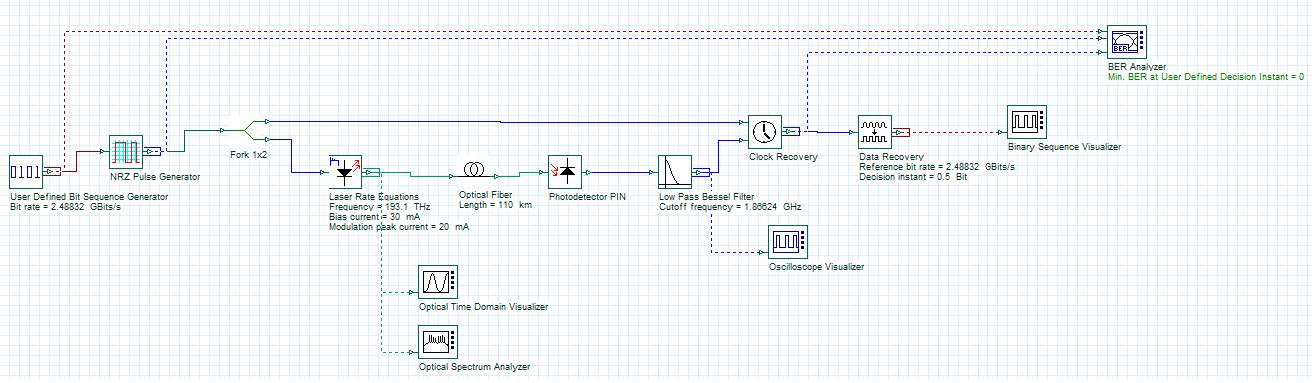# Matlab Cosimulation

Compatibility:

BER Analysis Using Matlab®/OptiSystem Automation

This example demonstrates the use of Matlab® software to call OptiSystem and calculate the bit error rate after the OptiSystem simulation.

Samples: MatlabOptiSysBER.m

The Matlab file “MatlabOptiSysBER.m” executes the following functions:

• Generates the data (sequences of bits) that are transmitted through OptiSystem;
• Opens OptiSystem;
• Setup the global parameters and transfer the binary data to OptiSystem;
• Run the simulation;
• Transfer the received data from OptiSystem to Matlab;
• Calculates the bit error rate (BER).Figure 1: System layout

To run this example, the user has to check if the path provided for the OpticalLinkProject.osd file is correct.